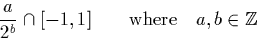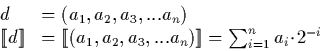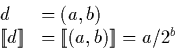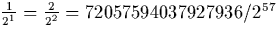Next: (Mantissa, Exponent) Representation Up: Representing Reals as Streams Previous: Signed Binary Digit Streams

## Dyadic Rational Streams

The second basic representation used is a stream of dyadic rationals in the range [-1,1]:Observe that there are a infinitely many such numbers. We can define algorithms for a number of simple operations and relationships on these dyadic rationals.

We treat these dyadic rationals as if they were digits, and from now on term them dyadic digits. Dyadic digits may be represented in several ways. One possibility is to use a datatype which is either a symbol representing one, minus one, or is an arbitrary but finite length string or binary digits representing a binary fraction. ie:Another alternative is to represent the digit as a pair of arbitrary length integers. ie.In the latter case, any given dyadic rational may be represented in several ways (eg.). One solution to the problem of having an unnecessarily large representation for a dyadic rational is to force it into its lowest terms by ensuring that zero is represented as (0,0), and that in all other cases the numerator a is odd and the denominator positive. This prevents the integers representing the dyadic digit swelling more than is strictly necessary.

The choice of internal representation used in the implementation is discussed in section 6.2.5, and we give algorithms for the basic operations using these digits alluded to above in appendix A.

We now define the dyadic digit stream representation in exactly the same way as we did the signed binary digit streams, except that instead of just using three digits -1,0,1, we use instead the dyadic digits described above.

Observe that like signed binary streams, the dyadic stream representation has a fixed rate of convergence. Dyadic digits, however, can incorporate significantly more information than signed binary digits. This can be extremely useful for simplifying certain algorithms, and can both improve or destroy the performance of certain calculations. The performance of the algorithms is discussed in detail in chapter 7.Next: (Mantissa, Exponent) Representation Up: Representing Reals as Streams Previous: Signed Binary Digit Streams
Martin Escardo
5/11/2000